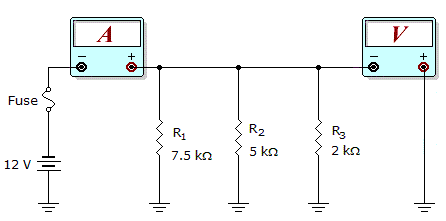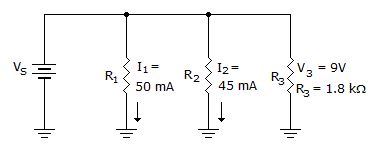# Electronics - Parallel Circuits

Exercise : Parallel Circuits - General Questions
26.What would these meter readings indicate about the circuit in the given circuit?

Meter Readings: I = 0 mA, V = 0 V

R1 is open.
R2 is open.
The fuse is open.
The circuit is operating normally.
Explanation:
No answer description is available. Let's discuss.

27.
If 550 mA of current leaves a node that had 250 mA entering from one branch, how much current would enter from the other?
250 mA
300 mA
550 mA
800 mA
Explanation:
No answer description is available. Let's discuss.

28.What would these meter readings indicate about the circuit in the given circuit?

Meter Readings: I = 10 mA, V = 12 V

R1 is open.
R2 is open.
The fuse is open.
The circuit is operating normally.
Explanation:
No answer description is available. Let's discuss.

29.What does RT equal in the given circuit?

901802001.8 k